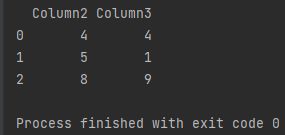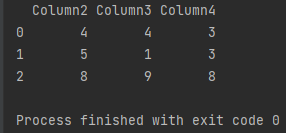Pandas How To Uncategorized How to select columns the Pandas way

# How to select columns the Pandas way

In this tutorial you will learn how to select columns in Pandas.

I will show you how to select columns using the functions provided by the Pandas library.

## How to select columns by index

Use the iloc function to select columns using indexes. Below shows how to select columns: second and third.

```import pandas as pd

my_df = pd.DataFrame({'id':['id1','id2','id3'],
'Column1': ['2', '7', '6'],
'Column2': ['4', '5', '8'],
'Column3': ['4', '1', '9'],
'Column4': ['3', '3', '8'],
'Column5': ['2', '5', '4'],
'Column6': ['2', '7', '3']})

selected_columns = my_df.iloc[:, 2:4]

print(selected_columns)

```## How to select columns by name

Use the loc function to select columns by their names. Below shows how to select columns from “Column2” to “Column4”.

```import pandas as pd

my_df = pd.DataFrame({'id':['id1','id2','id3'],
'Column1': ['2', '7', '6'],
'Column2': ['4', '5', '8'],
'Column3': ['4', '1', '9'],
'Column4': ['3', '3', '8'],
'Column5': ['2', '5', '4'],
'Column6': ['2', '7', '3']})

selected_columns = my_df.loc[:, 'Column2':'Column4']

print(selected_columns)
```There are many other ways to choose speakers, but these two are the most relevant to the Pandas package, which is what I’m passionate about and the Pandas How To page is dedicated to it.

Tags: ,

## How to calculate sum of rows and columns in PandasHow to calculate sum of rows and columns in Pandas

Here’s how to calculate sum of rows and columns in Pandas dataframe.

## How to print column namesHow to print column names

In today’s post you will learn how to print column names from your dataframe in Pandas.